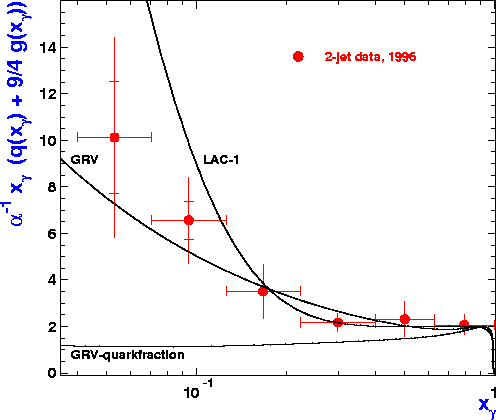Measurement of Parton distributions in the  Photon

The electron beam at HERA is a copious source of (quasireal) photons which are radiated off. These photons  have a high cross section to interact with the protons at high center of mass energies of about 200 GeV.  A recent study  thesis O. Kaufmann '98    has used hard scattering processes which lead to at least two  hard jets in the final state to measure the parton content of the photon especially the gluons.  The results are shown in the following figures:

The paper is now published:  dijet production in gamma-p interactions and photon structureeffective parton distribution in the photon

The effective parton distribution is shown as a function of the momentum fraction of the photon x which is carried by the parton. At large x  the distribution is dominated by quarks and antiquarks whereas at low x gluons dominate. The quark distribution shown as black curve is extracted from two photon measuerements at LEP.
If this known fraction is subtracted then we can determine the gluon momentum disribution in the photon as shown below.The new measurement ( red points) shows that the gluon distribution is significant a small x. The new measurement is in good agreement with less precise earlier measurements.  The black curves show different proposed parametrisations of the gluon distribution based on QCD fits to e+e- data.